Representations of Surfaces (part 2):  z=f(x,y)

In:=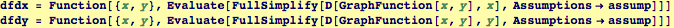Out=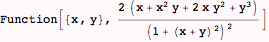Out=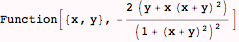This is the surface with lines of constant curvature superimposed, and with colors associated with the local normal.

In:=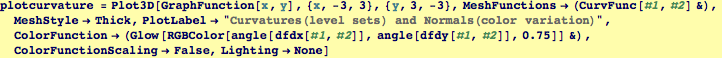Out=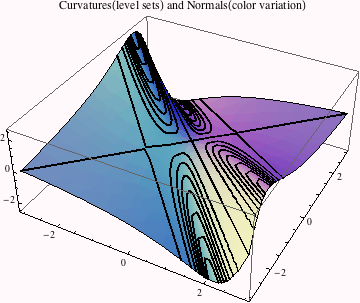Visualizing all the examples together.

In:=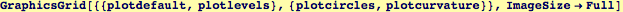Out=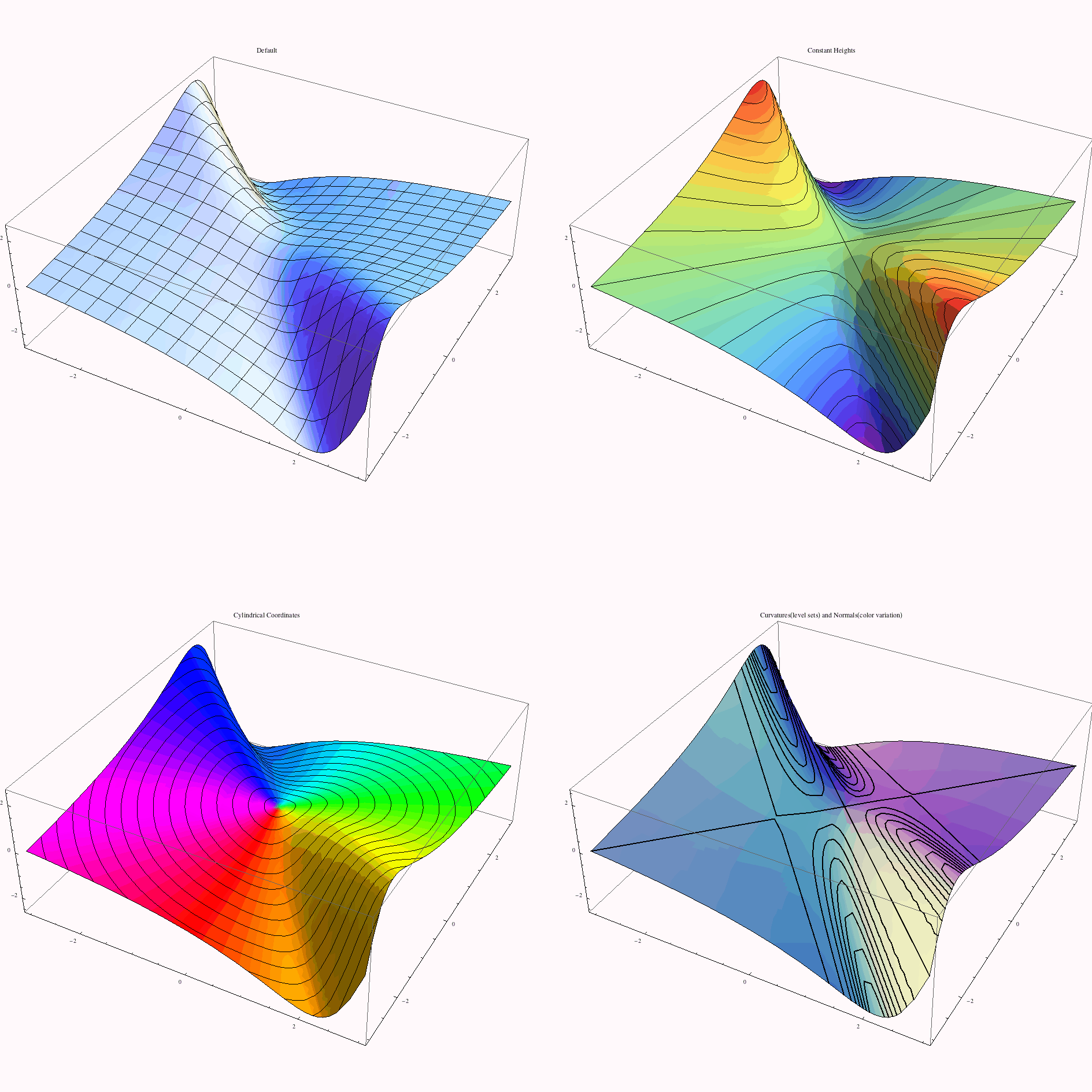Created by Wolfram Mathematica 6.0  (28 September 2007)